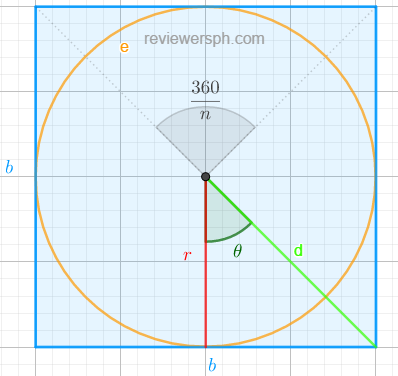### Math Notes

Subjects

#### Trigonometry Solutions

##### Topics || Problems

Show that the area of a regular polygon of $$n$$ sides circumscribed about a circle of radius $$r$$ is $$nr^2 \tan \frac{180^o}{n}$$

In a $$n$$ side regular polygon, there are exactly $$n$$ similar triangles whose base is on one side of the polygon. The sum of the area of these triangles $$(A_t)$$ is the area of the regular polygon, $$A_p$$.

$$A_p = nA_t$$

The angle of the triangle whose vertex is at the center of the circle can be calculated by $$\frac{360}{n}$$. Thus, $$\theta = \frac{360}{2n} = \frac{180}{n}$$.The area of the triangle, $$A_t = \frac{1}{2} b r$$

$$\tan \theta = \frac{b}{2r}$$

$$b = 2r \tan \theta$$

$$A_t = r^2 \tan \theta$$

Therefore, $$A_p = nr^2\tan \theta$$ but, $$\theta = \frac{180}{n}$$, thus $$A_p = nr^2\tan \frac{180}{n}$$